# Writing a simple Compiler on my own - Datatype attribute for Expressions [C][Flex][Bison][Custom Thumbnail]

All the Code of the series can be found at the Github repository:
https://github.com/drifter1/compiler

# Introduction

Hello it's a me again @drifter1! Today we continue with my Compiler Series, a series where we implement a complete compiler for a simple C-like language by using the C-tools Flex and Bison. In this article we will be adding and managing a new attribute for expressions, which is the "result" datatype. This will be needed for checking if assigning an expression or passing it as parameter is compatible with the identifier and parameter correspondingly. So, the next articles will be around that...

## The topics that we will cover today are:

1. Visualizing the Problem
2. Parameter datatype
3. Changes in AST Nodes and creation functions
4. Running the compiler

## Requirements:

Actually you need to read and understand all the topics that I covered in the series as a whole, as these articles will give you access to knowledge about:
• What Compiler Design is (mainly the steps)
• For which exact Language the Compiler is build for (Tokens and Grammar)
• How to use Flex and Bison
• How to implement a lexer and parser for the language using those tools
• What the Symbol Table is and how we implement it
• How we combine Flex and Bison together
• How we can pass information from the Lexer to the Parser
• How we define operator priorities, precedencies and associativity
• What Semantic Analysis is (Attributes, SDT etc.)
• How we do the so called "Scope Resolution"
• How we declare types and check the type inter-compatibility for different cases, including function parameters
• How we check function calls later on using a "Revisit queue", as function declarations mostly happen after functions get used (in our Language)
• Intermediate Code Representations, including AST's
• How we implement an AST (structure and management)
• Action Rules for AST nodes and more

## Difficulty:

Talking about the series in general this series can be rated:
Today's topic(s) can be rated:
• Medium

# Actual Tutorial Content

## Visualizing the Problem

When assigning an expression to an identifier or using an expression as a parameter in function calls, we need to have a way to know if the datatype of that expression is "compatible". The types of expressions that we have in our language can be visualized as following: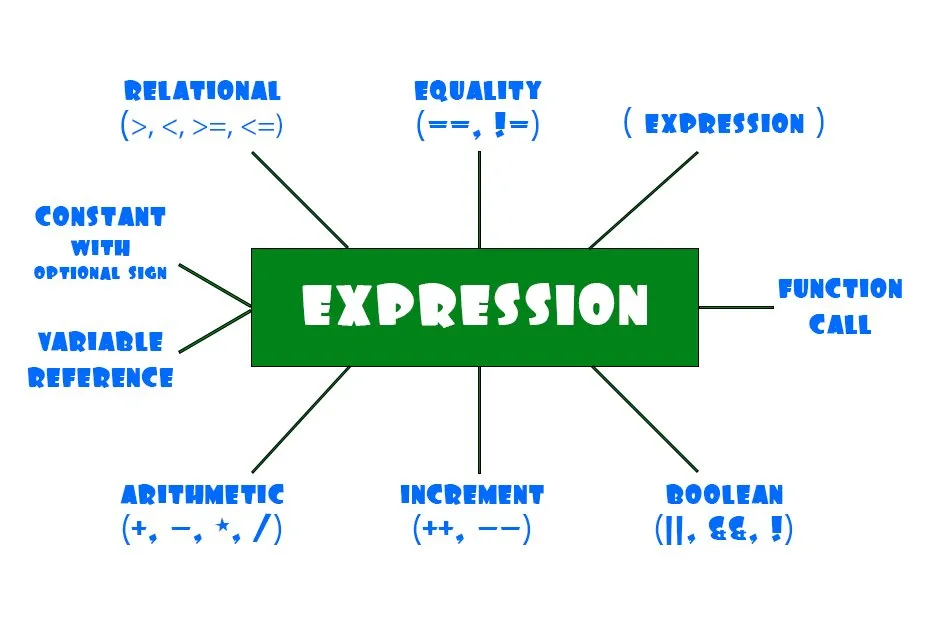Some of those expressions are operators, whilst others act as operands. The operands constant, variable and function call are of a specific datatype. Constants are of a specific constant type. The type of a variable is defined in it's declaration. The datatype of a function call is actually just the return type/value of the function. So, what's left to do now is give the operator nodes a new attribute so that we can store the result datatype of applying the operator on one or two operands. Of course an operator node can be used as an operand in other expressions and so on...

In general an "operator" expression looks as following: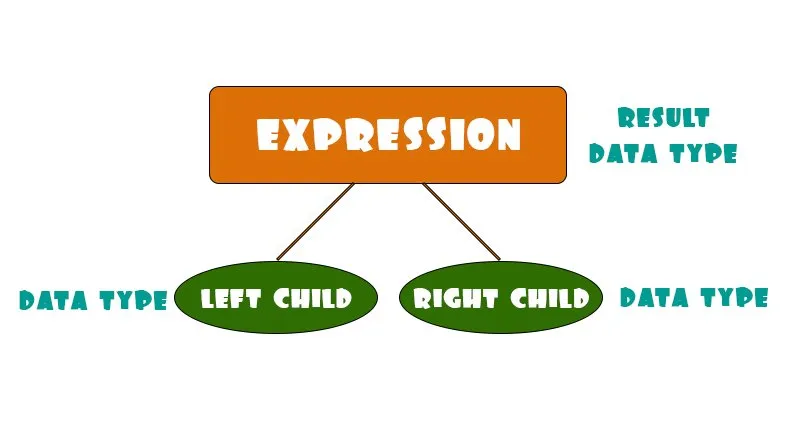So, each child has a specific type (right child can also be NULL for cases like the Boolean NOT). The parent expression node will store the result type of applying the operator on those two expressions/operands.

The special cases of having an actual operand as operand can be visualized as following: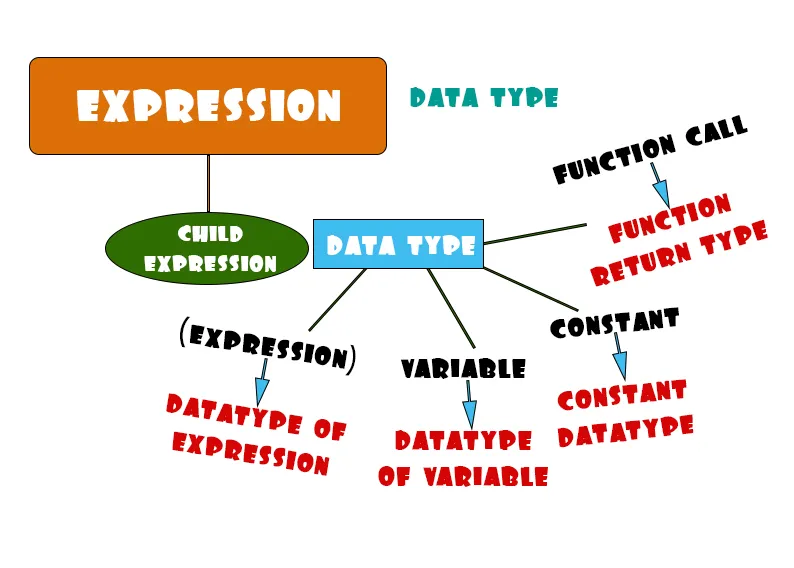When having an expression inside of parentheses we just push the information "higher". In the other cases we just pass the "constant type", "variable data type" or "function return type" as the type of the expression and so operand.

## Parameter datatype

In the articles where we did function declarations, and more specifically at the point where we defined parameters, we forgot to update the symbol table entry of the parameter identifier with the correct type. We only created and returned a parameter, without even touching the parameter's symbol table entry. So, what we have to do first (before we see errors out of nowhere) is go to the "parameter"-rule and set the type of the symbol table entry. Of course we should not forget the special cases of pointers and arrays. To distinguish them from "simple" variables we already set the "st_type" entry of the symbol table entry to the correct value of "POINTER_TYPE" and "ARRAY_TYPE", as it's passed by the "variable" non-terminal. So, the datatype inside of the "type"-rule will go to the "st_type" entry when talking about "simple" variables. For the special cases this datatype will be stored in the "inf_type" entry. This is all pretty similar to what we did back in variable declarations!

In Code this looks as following:
``````parameter : { declare = 1; } type variable
{
declare = 0;
// set type of symbol table entry
if(\$3->st_type == UNDEF){ /* "simple" type */
set_type(\$3->st_name, \$2, UNDEF);
}
else if(\$3->st_type == POINTER_TYPE){ /* pointer */
set_type(\$3->st_name, POINTER_TYPE, \$2);
}
else if(\$3->st_type == ARRAY_TYPE){ /* array  */
set_type(\$3->st_name, ARRAY_TYPE, \$2);
};
/* define parameter */
\$\$ = def_param(\$2, \$3->st_name, 0);
}
;``````

## Changes in AST Nodes and creation functions

As you might have guessed already, an actual new attribute of the name "data_type" only has to be added to operator nodes. In the rest of the nodes we are able to access the data type using the other entries and mainly the symbol table entry/item. So, Arithmetic, Boolean, Relational and Equality nodes are the only AST Nodes that will be affected. All of them will be extended with a new integer variable "data_type".

In Code this looks as following:
``````typedef struct AST_Node_Arithm{
enum Node_Type type; // node type
// data type of result
int data_type;
// operator
enum Arithm_op op;
struct AST_Node *left;  // left child
struct AST_Node *right; // right child
}AST_Node_Arithm;
typedef struct AST_Node_Bool{
enum Node_Type type; // node type
// data type of result
int data_type;
// operator
enum Bool_op op;
struct AST_Node *left;  // left child
struct AST_Node *right; // right child
}AST_Node_Bool;
typedef struct AST_Node_Rel{
enum Node_Type type; // node type
// data type of result
int data_type;
// operator
enum Rel_op op;
struct AST_Node *left;  // left child
struct AST_Node *right; // right child
}AST_Node_Rel;
typedef struct AST_Node_Equ{
enum Node_Type type; // node type
// data type of result
int data_type;
// operator
enum Equ_op op;
struct AST_Node *left;  // left child
struct AST_Node *right; // right child
}AST_Node_Equ;``````

We will not give this new type as a parameter, but manage the return data type inside of the creation function of each of those cases. To do this easily, we need a function that gives us this result data type. Luckily we already created a function with the name "get_result_type" for that, which is implemented inside of the "semantics.c" file. But, that's not enough! What about getting this type. There are lots of types of expressions and we might even add new ones later on. So, what do we do? Well, it's pretty simple, we just have to create a function that gives back the data type of an AST Node.

This function has to give back:
• The "data_type" attribute of Arithmetic, Boolean, Relational and Equality Nodes
• The "st_type" of the symbol table entry inside of an Increment Node (special case of arithmetic expression)
• The "st_type" or "inf_type" of the symbol table inside of an Reference Node, depending on if it's a "simple" variable or a special case of array or pointer
• The "const_type" of a Constant Node
• The "Return type" of a Function Call that is stored inside of the symbol table entry (we don't managed it yet and so we will return '1', just for testing purposes)
So, the Code is:
``````ast.h:
...
int expression_data_type(AST_Node *node);
---------------------------------------------------------------------
ast.c:
...
int expression_data_type(AST_Node *node){
/* temp nodes */
AST_Node_Arithm *temp_arithm;
AST_Node_Incr *temp_incr;
AST_Node_Bool *temp_bool;
AST_Node_Rel *temp_rel;
AST_Node_Equ *temp_equ;
AST_Node_Ref *temp_ref;
AST_Node_Const *temp_const;
AST_Node_Func_Call *temp_func_call;
/* return type depends on the AST node type */
switch(node->type){
case ARITHM_NODE: /* arithmetic expression */
temp_arithm = (AST_Node_Arithm *) node;
return temp_arithm->data_type;
break;
case INCR_NODE:   /* special case of increment */
temp_incr = (AST_Node_Incr *) node;
return temp_incr->entry->st_type;
break;
case BOOL_NODE:   /* boolean expression */
temp_bool = (AST_Node_Bool *) node;
return temp_bool->data_type;
break;
case REL_NODE:    /* relational expression */
temp_rel = (AST_Node_Rel *) node;
return temp_rel->data_type;
break;
case EQU_NODE:    /* equality expression */
temp_equ = (AST_Node_Equ *) node;
return temp_equ->data_type;
break;
case REF_NODE:    /* identifier reference */
temp_ref = (AST_Node_Ref *) node;
/* if "simple" type */
int type = temp_ref->entry->st_type;
if(type == INT_TYPE || type == REAL_TYPE || type == CHAR_TYPE){
return temp_ref->entry->st_type;
}
/* if array or pointer */
else{
return temp_ref->entry->inf_type;
}
break;
case CONST_NODE:  /* constant */
temp_const = (AST_Node_Const *) node;
return temp_const->const_type; /* constant data type */
break;
case FUNC_CALL:   /* function call */
temp_func_call = (AST_Node_Func_Call *) node;
return 1; /* just for testing */
return temp_func_call->entry->inf_type; /* return type */
break;
default: /* wrong choice case */
fprintf(stderr, "Error in node selection!\n");
exit(1);
}
}
...``````

Using this function we can now get the data type of any expression in our language. This means that the creation functions of the 4 operator nodes will use this and the result type function to set the entry of the data type. For Arithmetic, Relational and Equality nodes there is no special case of operator (the arithmetic operator INCR is managed by it's own node), whilst Boolean nodes have the special case of the NOT operator, which has only one operand.
In the end, the new creation functions are:
``````AST_Node *new_ast_arithm_node(enum Arithm_op op, AST_Node *left, AST_Node *right){
// allocate memory
AST_Node_Arithm *v = malloc (sizeof (AST_Node_Arithm));
// set entries
v->type = ARITHM_NODE;
v->op = op;
v->left = left;
v->right = right;
// set data type
v->data_type = get_result_type(
expression_data_type(left),  /* data type of left expression */
expression_data_type(right), /* data type of right expression */
ARITHM_OP                    /* operation type */
);
// return type-casted result
return (struct AST_Node *) v;
}
AST_Node *new_ast_bool_node(enum Bool_op op, AST_Node *left, AST_Node *right){
// allocate memory
AST_Node_Bool *v = malloc (sizeof (AST_Node_Bool));
// set entries
v->type = BOOL_NODE;
v->op = op;
v->left = left;
v->right = right;
// set data type
if(v->op != NOT){ /* AND or OR */
v->data_type = get_result_type(
expression_data_type(left),  /* data type of left expression */
expression_data_type(right), /* data type of right expression */
BOOL_OP                      /* operation type */
);
}
else{ /* NOT */
v->data_type = get_result_type(
expression_data_type(left), /* data type of left expression */
UNDEF,                      /* there is no right expression */
NOT_OP                      /* operation type */
);
}
// return type-casted result
return (struct AST_Node *) v;
}
AST_Node *new_ast_rel_node(enum Rel_op op, AST_Node *left, AST_Node *right){
// allocate memory
AST_Node_Rel *v = malloc (sizeof (AST_Node_Rel));
// set entries
v->type = REL_NODE;
v->op = op;
v->left = left;
v->right = right;
// set data type
v->data_type = get_result_type(
expression_data_type(left),  /* data type of left expression  */
expression_data_type(right), /* data type of right expression */
REL_OP                       /* operation type */
);
// return type-casted result
return (struct AST_Node *) v;
}
AST_Node *new_ast_equ_node(enum Equ_op op, AST_Node *left, AST_Node *right){
// allocate memory
AST_Node_Equ *v = malloc (sizeof (AST_Node_Equ));
// set entries
v->type = EQU_NODE;
v->op = op;
v->left = left;
v->right = right;
// set data type
v->data_type = get_result_type(
expression_data_type(left),  /* data type of left expression  */
expression_data_type(right), /* data type of right expression */
EQU_OP                       /* operation type */
);
// return type-casted result
return (struct AST_Node *) v;
}``````

Of course adding this new attribute I also tweaked the traversal and printing functions of nodes, that you can check out on GitHub.

## Running the compiler

Let's lastly run the Compiler for the "full_example.c" file...as always.

More specifically, let's check the datatype of the following 3 expressions:
``````...
else if(i == 5){
i = 2 * i;
...
}
else{
...
p = p + 1;
}
...
double add (double a, int b){
double res;
res = a + b + (-5);
return res;
}``````

Running the compiler we get: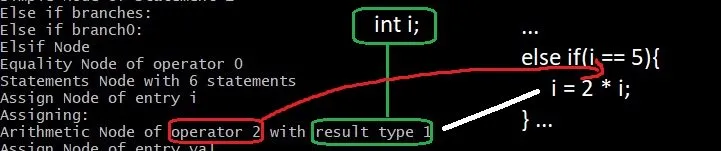...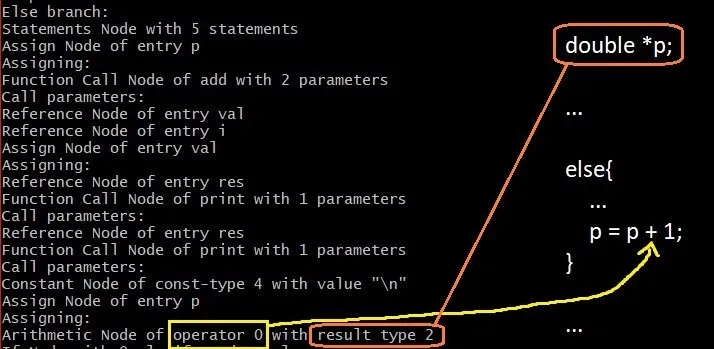...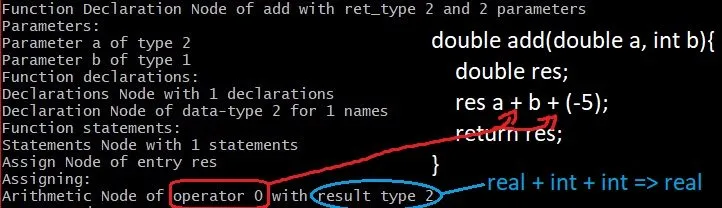You can see that the compiler truly managed to find the correct data type of those expressions. We might have to look back to this later on tho, as using a pointer, we are actually using it's pointing type right now, which is somewhat wrong. But, I think that you got the idea! :)

## RESOURCES

### References:

No references, just using code that I implemented in my previous articles.

### Images:

All of the images are custom-made!

## Final words | Next up on the project

And this is actually it for today's post! I hope that I explained everything as much as I needed to, meaning that you learned something out of it.

Next up on this series are:
• Semantic analysis (using even more action rules in Bison)
• Machine Code generation (MIPS Assembly)
Which are all topics that will need more than one article to complete. Also, note that we might also get into Optimizations later on, or could even extend the Language by adding complex datatypes (structs and unions), more rules etc.
So, see ya next time!

## GitHub Account:

https://github.com/drifter1Keep on drifting! ;)

H2
H3
H4
3 columns
2 columns
1 column